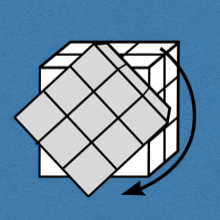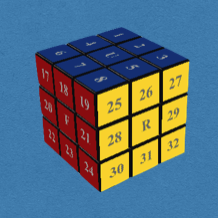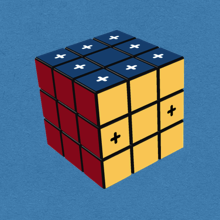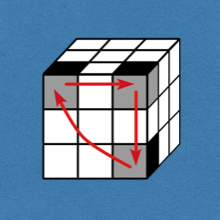## Rubik's Cube

It is rare to find a puzzle, or toy, that has captured the imagination of millions, is accessible to all age levels, is challenging, yet satisfying, and is so mathematically rich. Rubik's cube is one such puzzle.

The applet below was written by Werner Randelshofer ( CubeTwister).

In this course we'll investigate the cube, learn how to model it using group theory, uncover a solvability criteria, and best of all, we'll learn how to solve it by building our own move sequences.### Notation

We introduce the basic terminalogy and notation used for the cube.### Representation

We represent the cube as a subgroup of $S_{48}$ and compute with this group in SageMath.### Cubology

The Fundamental Theorem of Cubology: the solvability criteria for the cube.### Solve the Cube

Learn a basic 5-step solution for the cube. A perfect solution for beginners.